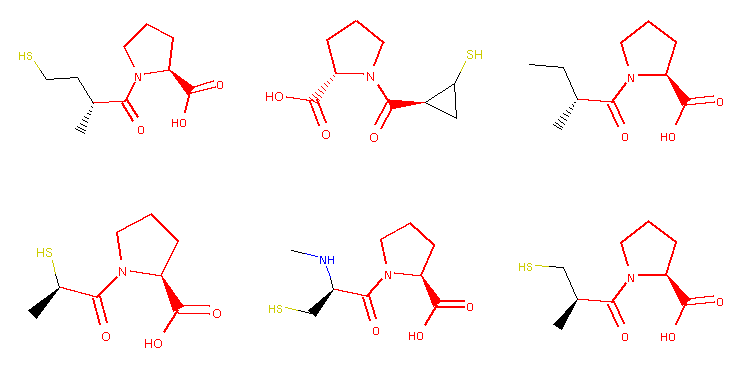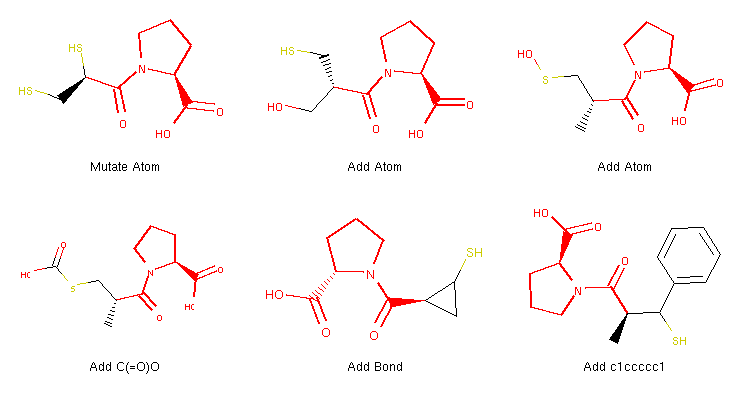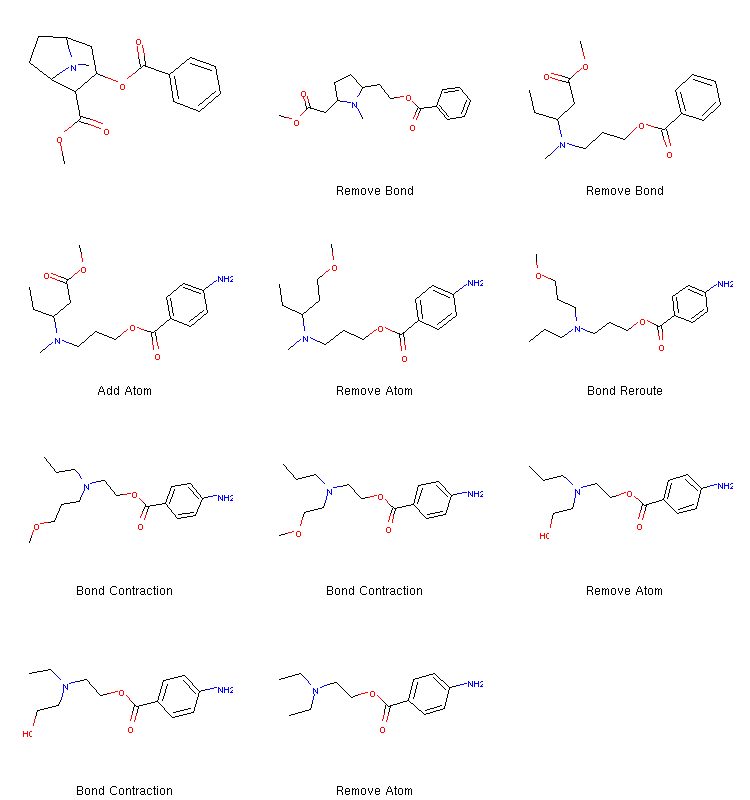## Usage Examples

This page features some code examples, which we hope are the main selling points of Molpher-lib. The library can generally do much more, though, and many of these examples are more thoroughly explained in the documentation.

### Generating Morphs

The main use case for Molpher-lib is automatic generation of new molecular structures from a given starting point. What 'derivatives' we get depends on a set of morphing operators. These operators can take any shape or form. In the original Molpher approach, they are a set of somewhat arbitrarily chosen structural modifications (add atom, add bond, remove atom, remove bond...), but they can also be elementary chemical transformations or other transformations that might be of interest.

Here is an example of how the library can be used to generate new structural analogs of captopril, a famous hypertension drug:

``````from rdkit import Chem
from molpher.core import MolpherMol
from molpher.core.morphing import Molpher
from molpher.core.morphing.operators import *

# define a collector -> a callback function that processes morphs as they are generated
strange_patterns = Chem.MolFromSmarts('[S,O,N][F,Cl,Br,I]')
sensible_morphs = dict()
def collect_sensible(morph, operator):
"""
simple collector, accepts morphs without some weird structural patterns
"""

rd_morph = morph.asRDMol()
if not rd_morph.HasSubstructMatch(strange_patterns):
sensible_morphs[morph.smiles] = morph

# load a molecule from SDF and generate some derived molecules with given morphing operators
mol = MolpherMol("captopril.sdf")
molpher = Molpher(
mol
, [ # list of morphing operators to use
, RemoveAtom()
, MutateAtom()
, RemoveBond()
, ContractBond()
, InterlayAtom()
, RerouteBond()
]
, attempts = 100 # create at most 100 molecules
, collectors = [collect_sensible]
)

# execute morphing and show created molecules
molpher()
as_mol_grid(sensible_morphs.values()) # draw generated structures in a grid``````
Sample code featuring the `Molpher` class. It demonstrates how a set of 'derivatives' can be formed from a source compound using various chemical operators implemented in Molpher-lib.
Generated image:Six morphs that were cropped out of the grid image depicting collected morphs. The image is generated with the `as_mol_grid` function (definition not part of the example), which also highlights locked atoms (in red).

You might notice that the typical '-pril' structural pattern is preserved among all of the generated structures in this example. It is because atoms forming this substructure were locked away from certain modifications in the `captopril.sdf` file. You can read how atom locking works in the introductory introductory tutorial where this example is from.

### Customized Operators

Since morphing operators play a crucial role in the generation process, the library makes implementations of new operators possible through the `MorphingOperator` interface:

``````from rdkit import Chem
from molpher.core import MolpherMol, MolpherAtom
from molpher.core.morphing import Molpher
from molpher.core.morphing.operators import *
from molpher.random import get_random_number

"""
Attaches a given molecule fragment to an atom in the molecule.
"""

def __init__(self, fragment, open_atoms_frag, oper_name):
self._name = oper_name # name of the operator
self._fragment = fragment # fragment as RDKit Mol
self._open_atoms_frag = open_atoms_frag # possible attachment positions on the fragment
self._orig_rdkit = None # original molecule as RDKit Mol
self._open_atoms = [] # possible attachment positions on the original molecule

def setOriginal(self, mol):
if self.original:
self._orig_rdkit = self.original.asRDMol()
self._open_atoms = []

for atm_rdkit, atm_molpher in zip(self._orig_rdkit.GetAtoms(), self.original.atoms):
free_bonds = atm_rdkit.GetImplicitValence()
self._open_atoms.append(atm_rdkit.GetIdx())

def morph(self):
combo_mol = Chem.EditableMol(Chem.CombineMols(
self._orig_rdkit
, self._fragment
))
atom_orig = self._open_atoms[get_random_number(0, len(self._open_atoms)-1)]
atom_frag = len(self.original.atoms) + self._open_atoms_frag[get_random_number(0, len(self._open_atoms_frag)-1)]
combo_mol = combo_mol.GetMol()
Chem.SanitizeMol(combo_mol)

ret = MolpherMol(other=combo_mol)
for atm_ret, atm_orig in zip(ret.atoms, self.original.atoms):

return ret

def getName(self):
return self._name

# define a collector -> a callback function that processes morphs as they are generated
strange_patterns = Chem.MolFromSmarts('[S,O,N][F,Cl,Br,I]')
sensible_morphs = dict()
def collect_sensible(morph, operator):
"""
simple collector, accepts morphs without some weird structural patterns
"""

rd_morph = morph.asRDMol()
if not rd_morph.HasSubstructMatch(strange_patterns):
sensible_morphs[morph.smiles] = morph
morph.parent_operator = operator.getName()

fragments = ['c1ccccc1', 'C(=O)O']
for frag in fragments:

# load a molecule from SDF and generate some derived molecules with given morphing operators
mol = MolpherMol("captopril.sdf")
molpher = Molpher(
mol
, [ # list of morphing operators to use
, RemoveAtom()
, MutateAtom()
, RemoveBond()
, ContractBond()
, InterlayAtom()
, RerouteBond()
, attempts = 100 # create at most 100 molecules
, collectors = [collect_sensible]
)

# execute morphing and show created molecules
molpher()
as_mol_grid(sensible_morphs.values()) # draw generated structures in a grid``````
Example code using the `MorphingOperator` abstract class to implement a customized operator `AddFragment`.
Generated image:Example structures of morphs produced with the code above.

This code is essentially the same as above, but contains a few more lines, which define the new operator itself. The created instances are used by the `Molpher` class in the same manner as the built-in operators.

When we focus on the definition of the operator itself, we can see that Molpher-lib can be easily integrated with the RDKit cheminformatics library because its `MolpherMol` instances can be cloned to RDKit molecules with a call to the `asRDMol` method.

Also note the improved collector information where we now set the `parent_operator` attribute of the generated morphs. The value of this attribute is then used to generate labels in the image and tells us what operator was used to generate the given structure. You can learn more about implementing operators in the appropriate section of the documentation

### Finding a Path

As was the case in the original Molpher approach, Molpher-lib is able to generate chemical space path from one molecule to another. Using the original Molpher algorithm from the algorithms package, we can perform a search from cocaine to procaine, for example:

``````from molpher.algorithms.classic.run import run
from molpher.algorithms.settings import Settings

# our source and target molecules
cocaine = 'CN1C2CCC1C(C(=O)OC)C(OC(=O)c1ccccc1)C2'
procaine = 'O=C(OCCN(CC)CC)c1ccc(N)cc1'

# directory where the path will be stored (as a pickled list)
storage_dir = 'data'

# initialize the exploration settings
settings = Settings(
source=cocaine
, target=procaine
, storage_dir=storage_dir
)

run(settings)``````
Code example illustrating how a path between two molecules (cocaine and procaine) can be generated.
The idea behind this approach is that structures on the chemical space path between these two molecules combine their structural features and could also be a basis of interesting pharmaceuticals.

### Implementing a Morphing Algorithm

If we want to have more control over what actually happens during the search process, we can use the exploration tree API to implement our own algorithm:

``````from molpher.core import ExplorationTree as ETree
from molpher.algorithms.functions import find_path

cocaine = 'CN1C2CCC1C(C(=O)OC)C(OC(=O)c1ccccc1)C2'
procaine = 'O=C(OCCN(CC)CC)c1ccc(N)cc1'

tree = ETree.create(source=cocaine, target=procaine) # create the tree
counter = 0
while not tree.path_found:
counter+=1
print("Iteration", counter)
tree.generateMorphs() # generate the first generation of morphs
tree.sortMorphs() # sort morphs according to their distance to target (ascending)
tree.filterMorphs() # remove molecules that do not meet certain criteria
tree.extend() # connect the remaining molecules to the exploration tree
tree.prune() # remove branches of the tree that do not converge

as_mol_grid(tree.fetchPathTo(tree.params['target']))``````
Sample code using the exploration tree API to implement the original algorithm from Molpher.
Generated path:Molecular structures on a chemical space path between cocaine and procaine.

This algorithm is basically an implementation of the one in the previous example. The tree is a data structure which keeps track of all possible paths that one might be interested in. We can extend the tree, remove certain molecules or paths and do many other things by performing operations. In the code example above, we used the shortcut methods available on the tree instance, but the built-in operations are all defined as separate callable classes under `molpher.core.operations`. Their behaviour can be adjusted using various settings, but it is also possible to define new operations end use them in a unified manner (see Defining Operations).

### Defining Operations

All operations that are already implemented in the library are available from the `molpher.core.operations` package. All of them share the same interface and can be performed on a tree using its `runOperation()` method (see the code example below). New operations can be easily implemented by inherirting from the `TreeOperation()` base class and implementing its `__call__()` method. In the example below, we show a simple filtering operation implementation:

``````from molpher.algorithms.functions import find_path
from molpher.core import ExplorationTree as ETree
from molpher.core.operations import *

cocaine = 'CN1[C@H]2CC[C@@H]1[C@@H](C(=O)OC)[C@@H](OC(=O)c1ccccc1)C2'
procaine = 'O=C(OCCN(CC)CC)c1ccc(N)cc1'

class NitorgenFilter(TreeOperation):

def __call__(self):
"""
This method can only be called when a tree is attached to the operation
(can be specified in the constructor, with the setTree() method or simply
by writing to the 'tree' attribute of the instance). When the runOperation()
method is executed, the tree is automatically added.
"""

new_mask = [ 'N' in x.smiles for x in self.tree.candidates ]

iteration = [
GenerateMorphsOper()
, SortMorphsOper()
, FilterMorphsOper() # the default filter
, CleanMorphsOper() # discards morphs that were previously filtered out
, NitorgenFilter() # our customized filter
, ExtendTreeOper() # connect the remaining structures to the tree
, PruneTreeOper()
]

tree = ETree.create(source=cocaine, target=procaine)
counter = 0
while not tree.path_found:
counter+=1
print("Iteration", counter)
for oper in iteration:
tree.runOperation(oper)

as_mol_grid(tree.fetchPathTo(tree.params['target']))``````
Example algorithm that uses a customized operation (`NitorgenFilter`) to discard molecules that do not contain nitrogen.

Every tree contains an array that masks the list of candidates that are currently evaluated (populated by `GenerateMorphsOper`). This mask is used to mark structures that should be removed from the list of candidates upon extending the tree or when `CleanMorphsOper` is called. Tree operations can be used to manipulate this mask and affect what molecules are accepted as the next generation in the evolution. Our customized operation in the example above does not really do much. It just discards generated structures that do not contain nitrogen. However, a more elaborate filtering scheme could also be implemented in this manner.

### More Examples

We have shown some common use cases of Molpher-lib. However, there is much more. For example, the library also provides means of traversing the molecules in the tree (or its subtree) or serializing tree snapshots at any point. You might want to head to the tutorial if you want a more complete overview of the software.